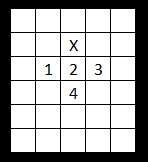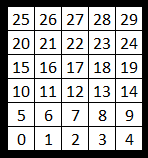# 'Fire' visual effect in JavaScript

The fire you're seeing above is a cool visual effect that was hot in the 1990s (pun intended).

It still looks great today and with very simple math and only about 20 lines of JavaScript, it's a great coding exercise.

In a nutshell, we create an array (matrix) of tiles. The higher the value of the tile, the brighter its color. In each animation frame, we randomize the tiles in the lowest visible row and for all the remaining tiles, we calculate their value based on the tiles below. More specifically, we first add the values of the three tiles immediately below and one tile two rows down:X is the tile being calculated and 1,2,3 and 4 are the tiles whose values are included in the calculation. Next, we divide the sum of the four tiles by a value slightly higher than 4, for example 4.1. This is what makes the flames die out as they go up.

We'll redraw all the tiles in each frame. To do this, we have to convert the array index value into screen coordinates. Because JS does not have 2-dimensional arrays, this conversion is a bit cumbersome.

Here are the tiles' indexes with an example array of 5 columns and 6 rows. The first tile is in the bottom left corner:The lowest row (elements 0-4) will always be empty.
The tiles in the second row from the bottom (elements 5-9) will be randomized in every frame to keep the fire going.
All the other rows (elements 10-29) will be calculated.
Here is the code with some more explanations. Enjoy!



Check out these programming tutorials:Domino effect in Blender PythonWrecking ball effect in Blender Python

3d fractal in Blender Python

Fractals in Excel

Your first program in JavaScript: you need 5 minutes and a notepad

Animated fractal in 32 lines of Javascript

Tutorial - interactive, animated sprites in JavaScript

PS. Many thanks to /u/inu-no-policemen for some great improvements to the code!